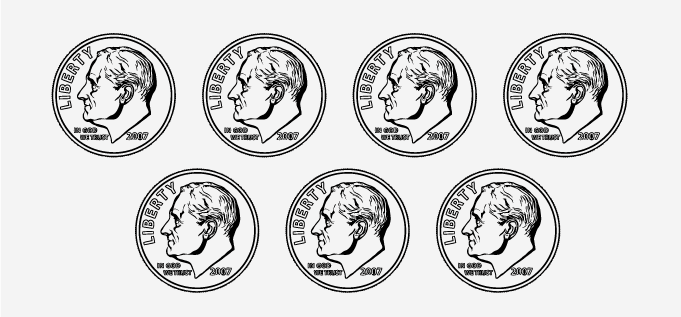# Multiplicative Inverse - Definition with Examples

The Complete K-5 Math Learning Program Built for Your Child

• 40 Million Kids

Loved by kids and parent worldwide

• 50,000 Schools

Trusted by teachers across schools

• Comprehensive Curriculum

Aligned to Common Core

## What is Multiplicative Inverse?

The word meaning of ‘inverse’ is something opposite in effect. So, the multiplicative inverse of a number is a number that nullifies the impact of the number to identity 1. Thus the multiplicative inverse of a number is a number by which the multiplication results in 1.

That is, number b is the multiplicative inverse of the number a, if a × b = 1.

For example: Here is a group of 7 dimes.To make them into groups of 1 each, we need to divide it by 7. The division is the reverse process of multiplication. Dividing by a number is equivalent to multiplying by the reciprocal of the number.

Thus, 7 ÷7=7 × 1=1.

Here, 17 is called the multiplicative inverse of 7. Similarly, the multiplicative inverse of 13 is 113.

Another word for multiplicative inverse is ‘reciprocal’. It comes from the Latin word ‘reciprocus’ which means returning.

## Multiplicative inverse of a natural number

In the given image to make the unit groups of 8 stars, we need to divide it by 8.8 ÷8=8 × 18=1

Thus, the multiplicative inverse of 8 is 18.

In general, if a is a natural number, the multiplicative inverse or reciprocal of a is 1a.

## Multiplicative inverse of a unit fraction

To make a unit fraction, say 1⁄4 to 1, we need to add it 4 times. Or in other words, multiply 14 by 4. Thus, the multiplicative inverse of 14 is 4.

In general, the multiplicative inverse or reciprocal of unit fraction 1x is x.

## Multiplicative inverse of a fraction

By what number should we multiply the fraction 34 to get 1?

34× ?=1

By the properties of equality, if we multiply or divide both sides of an equation by the same number the equation remains true. So, by multiplying the equation by 4 and dividing it by 3 on both sides gives us

3× ? × 4 ÷3 =1×4 ÷3

3× ? × 4343

Canceling the common terms:

1× ? = 43

Thus, the multiplicative inverse of 34 is  43.

The multiplicative inverse or reciprocal of a fraction ab is ba.

 Fun facts: The multiplicative inverse of zero is not defined. The multiplicative inverse of 1 is 1 itself.

Won Numerous Awards & Honors